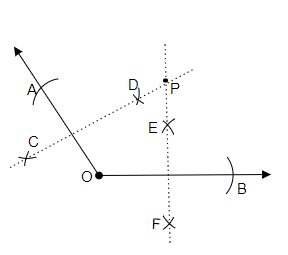# Draw any angle with vertex $O$. Take a point $A$ on one of its arms and $B$ on another such that $\mathrm{OA}=\mathrm{OB}$. Draw the perpendicular bisectors of $\overline{\mathrm{OA}}$ and $\overline{\mathrm{OB}}$. Let them meet at P. Is $\mathrm{PA}=\mathrm{PB}$ ?

To do:

We have to draw the perpendicular bisectors of $\overline{OA}$ and $\overline{OB}$, let them meet at $P$ and also find is $PA =PB$.

Solution:Steps of construction:

(i) Let us draw any angle with vertex $O$.

(ii) Now, by taking compasses let us draw an arc on one of its arm with $O$ as the centre and name the point of intersection of the arm with arc as $A$.

(iii) In the similar way let us draw another arc from $O$ with the compasses by taking a measure from $O$ to $A$ and name the point intersection of the arm with arc as $B$.

(iv) Now, by taking the measure greater than half of the length of $\overline{OA}$ let us draw two arcs above and below the $\overline{OA}$ and mark the point of intersections of the arcs as $C$ and $D$.

(v) In the similar way by taking the measure greater than half of the length of $\overline{OB}$ let us draw two arcs above and below the $\overline{OB}$ and mark the point of intersections of the arcs as $E$ and $F$.

(vi) Now let us join points $C$ and $D$ this forms the perpendicular bisector of $\overline{OA}$. In the similar way let us join points $E$ and $F$ this forms the perpendicular bisector of $\overline{OB}$.

(vii) By extending both the perpendicular bisectors we get a point of intersection let us point the point of intersection as $P$.

(viii) Now let us measure the length of $\overline{PA}$ and $\overline{PB}$ they are of equal length. Therefore, $\overline{PA}= \overline{PB}$.

Updated on: 10-Oct-2022

32 Views